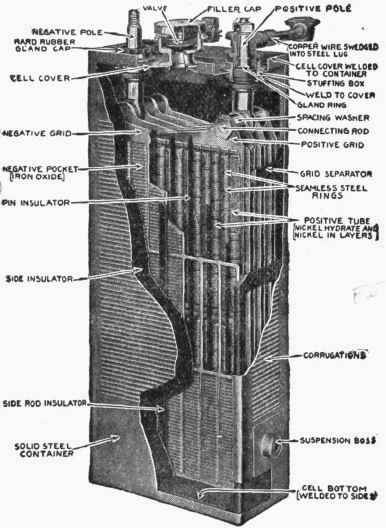A group of cells or electrical apparatus may be arranged in different ways. The wire from the zinc of the first cell may be connected to the carbon of the second, etc. (Fig. 77), or the wire from the zinc of the first may be connected to the zinc of the second, and the wire from the carbon of the first to the carbon of the second, and so on. (Fig. 78.)Fig. 76. - One Form of Storage Battery.

A battery is rated commercially by the resistance, and by the electromotive force of a single cell. There are two resistances to be considered in the calculations of the capacity of batteries: the resistance of the battery, due to polarization, etc., which is represented by R, and the resistance of the external circuit, such as the wire, which is designated by r. The current given by a battery - according to Ohm's Law - is equal to the electromotive force divided by the resistance, which in this case is divided into two parts.

Current = Electromotive force / Resistance (internal) + resistance (external)

C = E / R + r